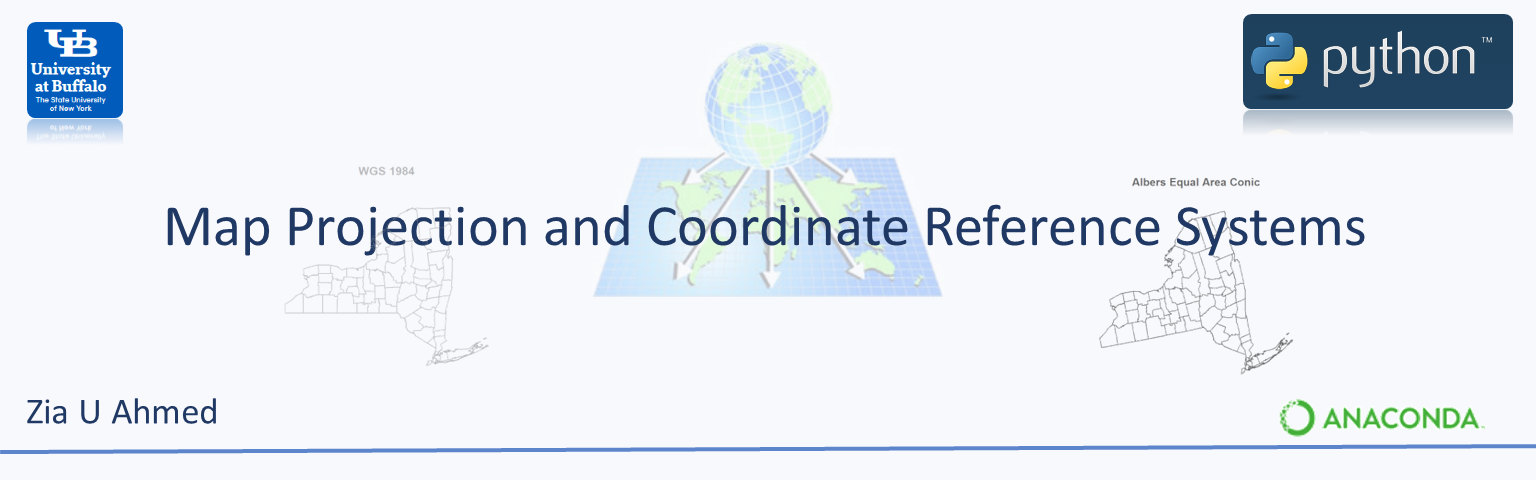Map projections is a systematic transformation a location on the surface of the earth or a portion of the earth (sphere or an ellipsoid) into on a plane (flat piece of paper or computer screen).

The coordinate reference system (CRS) or spatial reference system (SRS) is a coordinate based system used to locate features on the earth.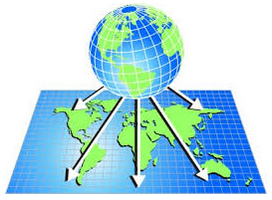In this section., we will cover following topics:

Data for this lesson could be found here

#### Geographic coordinate system (GCS)¶

Geographic coordinate systems use latitude and longitude to measure and locate feature on the globe. The GCS defines a position as a function of direction and distance from a center point of the globe, where the units of measurement are degrees. Any location on earth can be referenced by a point with longitude and latitude coordinates. For example, below figure shows a geographic coordinate system where a location is represented by the coordinate's longitude 80 degree East and latitude 55 degree North.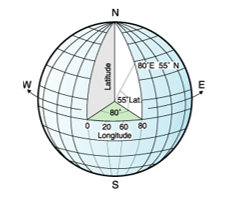The equidistant lines that run east and west each have a constant latitude value called parallels. The equator is the largest circle and divides the earth in half. It is equal in distance from each of the poles, and the value of this latitude line is zero. Locations north of the equator has positive latitudes that range from 0 to +90 degrees, while locations south of the equator have negative latitudes that range from 0 to -90 degrees.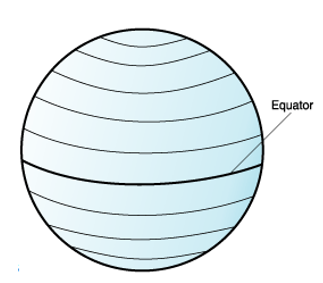The lines that run through north and south each have a constant longitude value and form circles of the same size around the earth known as meridians. The prime meridian is the line of longitude that defines the origin (zero degrees) for longitude coordinates. One of the most commonly used prime meridian locations is the line that passes through Greenwich, England. Locations east of the prime meridian up to its antipodal meridian (the continuation of the prime meridian on the other side of the globe) have positive longitudes ranging from 0 to +180 degrees. Locations west of the prime meridian has negative longitudes ranging from 0 to -180 degrees.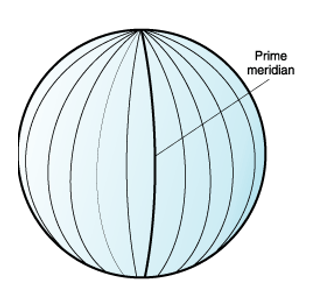The latitude and longitude lines cover the globe to form a grid that know as a graticule. The point of origin of the graticule is (0,0), where the equator and the prime meridian intersect. The equator is the only place on the graticule where the linear distance corresponding to one degree latitude is approximately equal the distance corresponding to one degree longitude. Because the longitude lines converge at the poles, the distance between two meridians is different at every parallel.

#### The World Geodetic system 84¶

The most recent geographic coordinate system is the World Geodetic system 84 also known as WGS 1984 or EPSG:4326 (EPSG- European Petroleum Survey Group). It consists of a standard coordinate system, spheroidal reference (the datum or reference ellipsoid) and raw altitude.

#### Projected coordinate system¶

A projected coordinate system provide mechanisms to project maps of the earth's spherical surface onto a two-dimensional Cartesian coordinate (x,y coordinates) plane. Projected coordinate systems are referred to as map projections. This approach is useful where accurate distance, angle, and area measurements are needed. The term 'projection' is often used interchangeably with projected coordinate systems.

Commonly use projected coordinate systems include:

• Universal Transverse Mercator

• Albers Equal Area Conic

#### Universal Transverse Mercator¶

The most widely used two-dimensional Cartesian coordinate system is the Universal Transverse Mercator (UTM) system which represents a horizontal position on the globe and can be used to identify positions without having to know their vertical location on the 'y' axis. The UTM system is not a single map projection. It represents the earth as sixty different zones, each composed of six-degree longitudinal bands, with a secant transverse Mercator projection in each. The UTM system divides the Earth between 80 S and 84 N latitude into 60 zones, each 6 of longitude in width. Zone 1 covers longitude 180 to 174 W; zone numbering increases eastward to zone 60, which covers longitude 174 to 180.